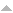# Effects of population and population pressure on forest resources and their conservation: a modeling study

 Title Effects of population and population pressure on forest resources and their conservation: a modeling study Publication Type Journal Article Year of Publication 2014 Authors Misra A.K., Lata K, Shukla J.B. Journal Environ Dev Sustain Volume 16 Start Page 361 Keywords Forest resource, Mathematical model, Population, Population pressure, Stability A. Abstract In this paper, a nonlinear mathematical model is proposed and analyzed to study the depletion of forest resources caused by population and the corresponding population pressure. It is assumed that the cumulative density of forest resources and the density of populations follow logistic models with prey–predator type nonlinear interaction terms. It is considered that the carrying capacity of forest resources decreases by population pressure, the main focus of this paper. A conservation model is also proposed to control the population pressure by providing some economic incentives to people, the amount of which is assumed to be proportional to the population pressure. The model is analyzed by using stability theory of differential equations and numerical simulation. The model analysis shows that as the density of population or population pressure increases, the cumulative density of forest resources decreases, and the resources may become extinct if the population pressure becomes too large. It is also noted that by controlling the population pressure, using some economic incentives, the density of forest resources can be maintained at an equilibrium level, which is population density dependent. The simulation analysis of the model confirms analytical results. Source Document Accessible with appropriate permission
Displaying 1 - 1 of 1 records found.
TitleType

### Effects of population and population pressure on forest resources and their conservation: a modeling study

Model
in this model, cumulative density of forest resources and population density use generalized logistic models with predatory-prey type nonlinear interactions. The model is an effort to capture not only the effect of population density on forest resources (a logistic model), but also the effect of population pressure on forest resources, which grows proportionally to population density and is limited by the carrying capacity of the forest.  Additionally, it models the effect of economic...
01 Oct 2016